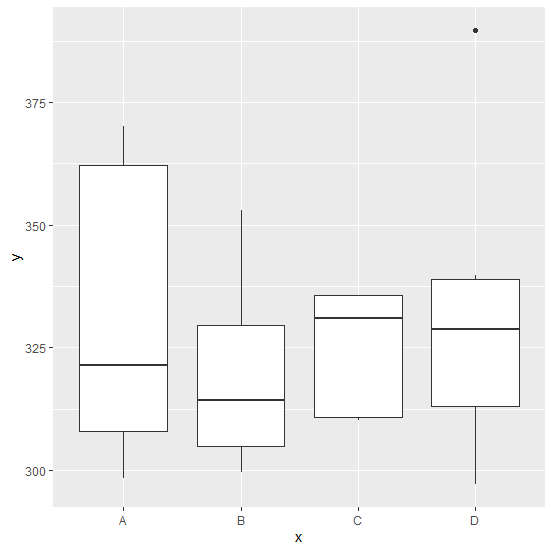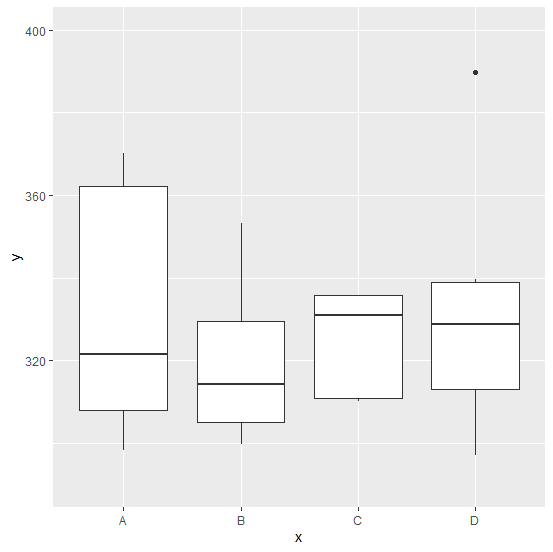# How to change the Y axis limit for boxplot created by using ggplot2 in R?

One of the most important aspects of a boxplot is Y-axis labels because these labels help us to understand the limit of the variable. Since R generate these labels automatically in a good way, we stick with that but we can change that using coord_cartesian function with ylim as shown in the below example.

## Example

Live Demo

Consider the below data frame −

set.seed(1212)
x<-sample(LETTERS[1:4],20,replace=TRUE)
y<-rnorm(20,330,24)
df<-data.frame(x,y)
df

## Output

   x    y
1 D 339.7344
2 B 321.6099
3 A 362.2010
4 A 370.1631
5 D 336.1964
6 B 306.7497
7 D 321.2534
8 D 297.1067
9 C 330.8902
10 B 353.0927
11 A 308.0316
12 C 335.7435
13 A 321.4714
14 B 299.6519
15 D 389.7760
16 A 298.2838
17 C 335.7696
18 C 310.1602
19 D 310.3076
20 C 310.8022

## Example

library(ggplot2)
ggplot(df,aes(x,y))+geom_boxplot()

## OutputCreating boxplot of y with different limits on the Y-axis −

ggplot(df,aes(x,y))+geom_boxplot()+coord_cartesian(ylim=c(290,400))

## Output